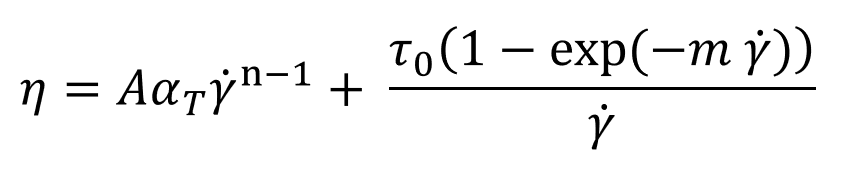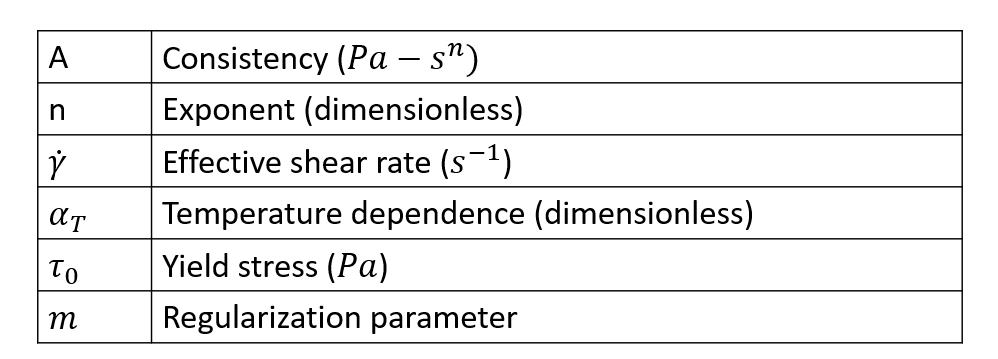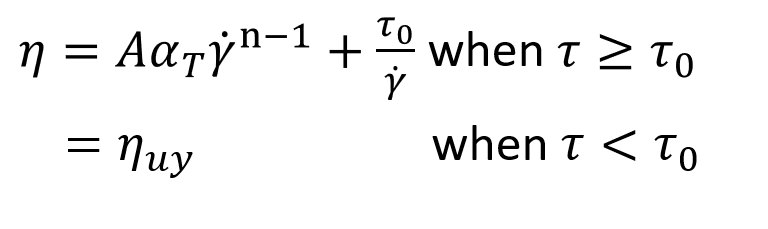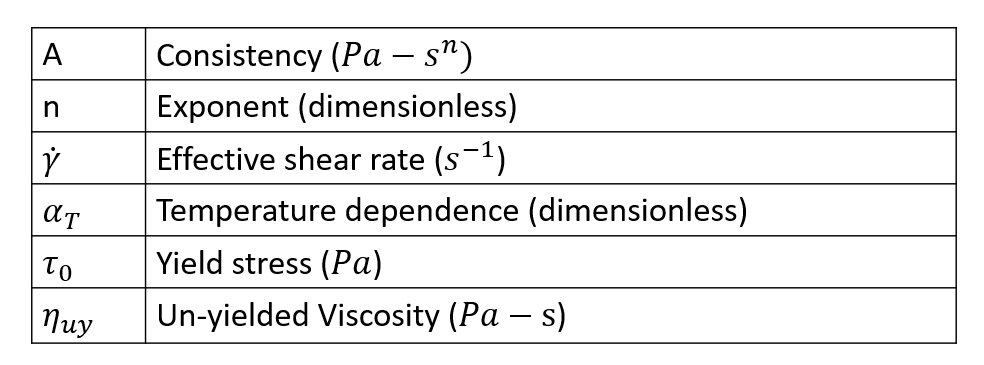# Herschel Bulkley Model

Regularized Herschel-Bulkley model is a four-parameter model and it is given by the following equation.This model describes the viscosity using a power-law relationship and a yield criterion. The exponent n determines the nature of the power law relationship and the yield criterion is implemented using the regularized model with yield stress t0 and regularization parameter m. Higher the value of m, closer the approximation to the actual yield stress behavior of Herschel-Bulkley model. For practical purposes, value of m=10000 should be suffice. This regularization parameter controls the exponential growth of stress. The original Herschel-Bulkley model, which is given by the following equation, suffers from the disadvantage of stress growing very large when the shear rate tends to zero. This will lead to numerical instability and also increase the difficulty of obtaining a converged solution. Hence, this model is regularized using the parameter m.In addition to the above data, few additional parameters arise due the temperature dependence of material data; these variables are discussed in Section titled Temperature Dependence.

## Syntax

Syntax of the data packet Polymer is as follows:

 Polymer PolymerName { ConstitutiveModel = "Cross" Density = ρ SpecificHeat = Cp (T) Conductivity = K (T) CoeffOfThermalExpansion βT Volumetric Heat Source = Qvol TimeConstant = Α Exponent = n YieldStress = τ0 StressExponentGrowth= m TemperatureDependence = "None" }

Parameter Description Units Data Type Condition Typical Value
ConstitutiveModel Describes the model used None String Required "HerschelBulkely"
Density Density of the polymer kg/m^3 Constant Required 995.0
SpecificHeat Specific heat at constant pressure J/kg/K Constant/F(T) Required 2000.0
Conductivity Thermal conductivity W/m?k Constant/F(T) Required 0.167
CoeffOfThermalExpansion Indicates the change in volume with change in temperature 1/K Constant Required 1.0e-05
VolumetricHeatSource Heat generated/removed in the volume by methods like elctrical heating W/m63 Constant Required 0.0
Consistency One of the parameters of the Herschel—Bulkley model. When n=1 it is same as viscosity Pa Constant Required 0.01
Exponent Power law index, defines the dependency of viscosity on shear rate. None Constant Required 0.66
ZeroShearRateLimit . See Power law model 1/s Constant Required 0.01
YieldStress Oneo f the parameters of the Herschel-Bulkley model. Pa Constant Required 1.0E+05
StressExponentGrowth One of the parameters of the Herschel—Bulkley model. It is also known as the regularization parameter. It controls the exponential growth of stress s Constant Required
TemperatureDependence See temperature Dependence None string Required "WLF"
ReferenceTemeprature Temperature at which data is calculated for the initialization step. K Constant Required only if TD is not "None" 533
FreezeTemperature This is the no flow temperature. Below this temperature, material ceases to flow. K Constant Required only if TD is not "None" 350
ActivationEnergy A parameter required by Arrhenius model. J/mol Constant Required only if Td is Exp(Q/RT) 16628
UniversalGasConstant A parameter from state equation PV = nRT, R is universal gas constant J/mol/K Constant Required onlyh if TD is Exp (Q/RT) 8.314
TemperatureSensitivity A derived parameter which has the same physical meaning as Q/R. K Constant Required only if TD is Exp(Tb/T) 2000 K
WLFConstant1 Constant C1 of WLF model none Constant Required only if TD is WLF 17.44
WLFConstant2 Constant C2 of WLF model. This is like DeltaT, hence the value is same in K and Celsius. K Constant Required only if TD is WLF 51.6
GlassTransitionTemperature Temperature below with polymer molecules ceases to move (frozen). There are few definitions of this term. K Constant Required only if TD is WLF 320
Beta Parameter in the relationship Exp(-Beta(DeltaT)) None Constant Required only if TD is exp(-Beta(Delta T) 0.005

F(T) - Function of Temperature. Can be specified as a TABLE1 or TCL function.

TD - TemperatureDependence